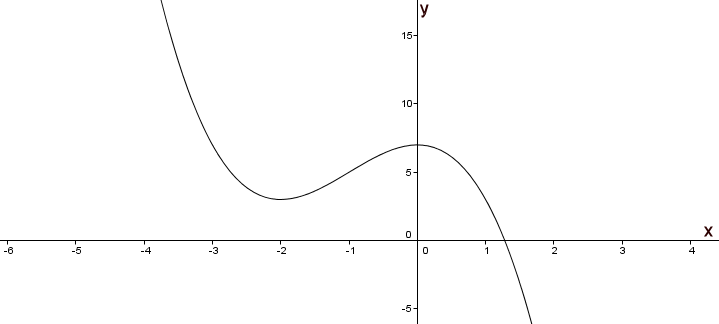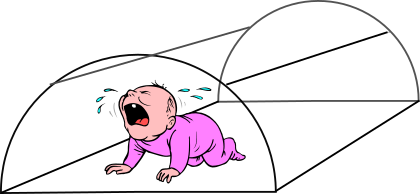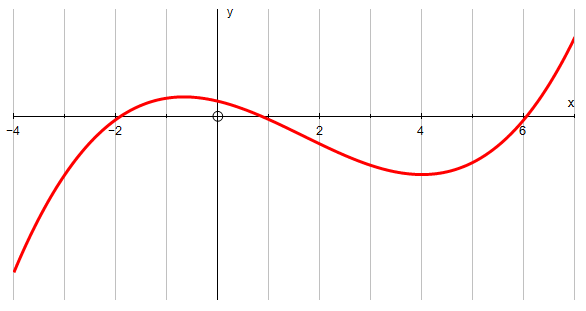# Exam-Style Questions on Differentiation

## Problems on Differentiation adapted from questions set in previous Mathematics exams.

### 1.

IB Studies

A function is given as $$f(x)=3x^2-6x+4+\frac3x,-2\le x \le 4, x\ne 0$$.

(a) Find the derivative of the function.

(b) Find the coordinates of the local minimum point of $$f(x)$$ in the given domain using your calculator.

### 2.

IB Studies

Consider the graph of the function $$f(x)=7-3x^2-x^3$$(a) Label the local maximum as A on the graph.

(b) Label the local minimum as B on the graph.

(c) Write down the interval where $$f(x)>5$$.

(d) Draw the tangent to the curve at $$x=-3$$ on the graph.

(e) Write down the equation of the tangent at $$x=-3$$.

### 3.

IB Studies

A child's play tent is made in the shape of half a cylinder. It is constructed from a fibreglass frame with material pulled tightly around it. The fibreglass frame consists of a rectangular base, two semi-circular ends and two further support rods, as shown in the following diagram.The semicircular ends each have radius $$r$$ and the support rods each have length $$d$$.

Let F be the total length of fibreglass used in the frame of the play tent.

(a) Write down an expression for $$F$$ in terms of $$r$$, $$d$$ and $$\pi$$.

The volume of the play tent is 0.95 m3.

(b) Write down an equation for the volume of the play tent in terms of $$r$$, $$d$$ and $$\pi$$.

(c) Show that $$F = 2\pi r + 4r + \frac{7.6}{\pi r^2}$$

(d) Find $$\frac{dF}{dr}$$

The play tent is designed so that the length of fibreglass used in its frame is a minimum.

(e) Find the value of $$r$$ for which $$F$$ is a minimum.

(f) Calculate the value of $$d$$ for which $$F$$ is a minimum.

(g) Calculate the minimum value of $$F$$.

### 4.

IB Studies

A package is in the shape of a cuboid and has a length $$l$$ cm, width $$w$$ cm and height of 12 cm.

(a) Express the volume of the package in terms of $$l$$ and $$w$$.

The total volume of the package is 2400 cm3.

(b) Show that $$l=\frac{200}{w}$$.

The package is tied up using a length of red string that fits exactly around the package in two different directions, as shown in the following diagram (not to scale).(c) Show that the length of string, $$x$$cm, required to tie up the package can be written as $$24+4w+\frac{400}{w}$$

(d) Sketch the graph of $$x$$ for $$0\lt w \le 12$$, clearly showing the local minimum point.

(e) Find $$\frac{dx}{dw}$$.

(f) Find the value of $$w$$ for which $$x$$ is a minimum.

(g) Find the value, $$l$$, of the package for which the length of string is a minimum.

(h) Find the minimum length of string required to tie up the package.

### 5.

IB Studies

Consider the function $$f(x)=6 - ax+\frac 3{x^2},x\neq 0$$

(a) Write down the equation of the vertical asymptote of the graph of $$y=f(x)$$.

(b) Write down $$f'(x)$$

The line T is the tangent to the graph of $$y=f(x)$$ at the point where $$x=1$$ and it has a gradient of -8.

(c) Show that $$a=2$$.

(d) Find the equation of T.

(e) Using your calculator find the coordinates of the point where the graph of $$y=f(x)$$ intersects the x-axis.

(f) The line T also intersects $$f(x)$$ when $$-2\le x\le 0$$. Find the coordinates of this intersection.

### 6.

IB Standard

If $$f(x)=xsinx$$, for $$-3\le x\le3$$

(a) Find $$f'(x)$$

(b) Sketch the graph of $$y=f'(x)$$, for $$-3\le x\le3$$

### 7.

IB Analysis and Approaches

Consider the cubic function $$f(x)=\frac{1}{6}x^3-2x^2+6x-2$$

(a) Find $$f'(x)$$

The graph of $$f$$ has horizontal tangents at the points where $$x = a$$ and $$x = b$$ where $$a < b$$.

(b) Find the value of $$a$$ and the value of $$b$$

(c) Sketch the graph of $$y = f'(x)$$.

(d) Hence explain why the graph of $$f$$ has a local maximum point at $$x = a$$.

(e) Find $$f''(b)$$.

(f) Hence, use your answer to part (e) to show that the graph of $$f$$ has a local minimum point at $$x = b$$.

(g) Find the coordinates of the point where the normal to the graph of $$f$$ at $$x = a$$ and the tangent to the graph of $$f$$ at $$x = b$$ intersect.

### 8.

IB Studies

Consider the function $$f(x)=x^3-9x+2$$.

(a) Sketch the graph of $$y=f(x)$$ for $$-4\le x\le 4$$ and $$-14\le y\le 14$$ showing clearly the axes intercepts and local maximum and minimum points. Use a scale of 2 cm to represent 1 unit on the x-axis, and a scale of 1 cm to represent 2 units on the y-axis.

(b) Find the value of $$f(-1)$$.

(c) Write down the coordinates of the y-intercept of the graph of $$f(x)$$.

(d) Find $$f'(x)$$.

(e) Find $$f'(-1)$$

(f) Explain what $$f'(-1)$$ represents.

(g) Find the equation of the tangent to the graph of $$f(x)$$ at the point where x is –1.

R and S are points on the curve such that the tangents to the curve at these points are horizontal. The x-coordinate of R is $$a$$ , and the x-coordinate of S is $$b$$ , $$b \gt a$$.

(h) Write down the value of $$a$$ ;

(i) Write down the value of $$b$$.

(j) Describe the behaviour of $$f(x)$$ for $$a \lt x \lt b$$.

### 9.

IB Standard

Let $$f(x)=\frac{g(x)}{h(x)}$$, where $$g(3)=36$$, $$h(3)=12$$, $$g'(3)=10$$ and $$h'(3)=4$$. Find the equation of the normal to the graph of $$f$$ at $$x=3$$.

### 10.

IB Analysis and Approaches

The displacement, in millimetres, of a particle from an origin, O, at time t seconds, is given by $$s(t) = t^3 cos t + 5t sin t$$ where $$0 \le t \le 5$$ .

(a) Find the maximum distance of the particle from O.

(b) Find the acceleration of the particle at the instant it first changes direction.

### 11.

IB Standard

The function $$f$$ is defined as follows:

$$f(x)=\frac{122}{1+60e^{-0.3x}}$$

(a) Calculate $$f(0)$$.

(b) Find a value of $$x$$ for which $$f(x)=85$$

(c) Find the range of $$f$$.

(d) Show that:

$$f'(x)=\frac{2196e^{-0.3x}}{(1+60e^{-0.3x})^2}$$

(e) Find the maximum rate of change of $$f$$.

### 12.

IB Standard

Let $$f(x)=jx^3+jx^2+kx+m$$ where $$j, k$$ and $$m$$ are constants.

(a) Find $$f'(x)$$

(b) If $$f'(x)\ge 0$$, show that $$j^2\le 3jk$$.

### 13.

IB Standard

The following diagram shows part of the graph of $$y=f (x)$$The graph has a local maximum where $$x=- \frac23$$, and a local minimum where $$x=4$$.

sketch the graph of $$y=f'(x)$$ for $$-4\le x \le 7$$

Write down the following in order from least to greatest: $$f(2),f'(4)$$ and $$f''(4)$$.

### 14.

A-Level

The following equation defines a curve which passes through $$A( 2 \pi ,3 \pi)$$

$$\sin 2y + y \cos^22x = x + c$$

(a) Find the exact value of the constant $$c$$.

(b) Find an expression for $$\frac{dy}{dx}$$

(c) Find the equation of the normal to the curve at A, giving your answer in the form $$y = mx + b$$

### 15.

IB Studies

Consider the function $$f(x)=\frac{20}{x^2}+kx$$ where $$k$$ is a constant and $$x\neq0$$.

(a) Write down $$f'(x)$$

The graph of $$y = f(x)$$ has a local minimum point at $$x=2$$.

(b) Show that $$k=5$$.

(c) Find $$f(1)$$.

(d) Find $$f'(1)$$.

(e) Find the equation of the normal to the graph of $$y=f(x)$$ at the point where $$x=1$$
Give your answer in the form $$ay+bx+c=0$$ where $$a, b, c \in \mathbb{Z}$$

(f) Sketch the graph of $$y=f(x)$$ , for $$-5\le x\le 10$$ and $$-10\le y\le 50$$.

(g) Write down the coordinates of the point where the graph of $$y=f(x)$$ intersects the x-axis.

(h) State the values of $$x$$ for which $$f(x)$$ is decreasing.

The exam-style questions appearing on this site are based on those set in previous examinations (or sample assessment papers for future examinations) by the major examination boards. The wording, diagrams and figures used in these questions have been changed from the originals so that students can have fresh, relevant problem solving practice even if they have previously worked through the related exam paper.

The solutions to the questions on this website are only available to those who have a Transum Subscription.

Exam-Style Questions Main Page

Search for exam-style questions containing a particular word or phrase:

To search the entire Transum website use the search box in the grey area below.